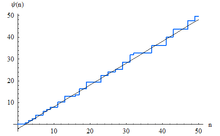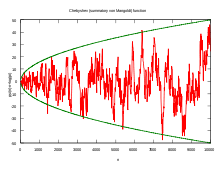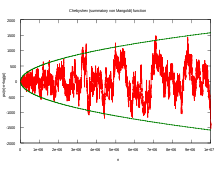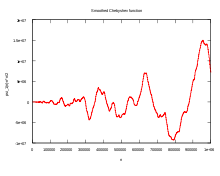# Chebyshev function

﻿
Chebyshev functionThe Chebyshev function ψ(x), with x < 50The function ψ(x) − x, for x < 10,000The function ψ(x) − x, for x < 10 million

In mathematics, the Chebyshev function is either of two related functions. The first Chebyshev function ϑ(x) or θ(x) is given by$\vartheta(x)=\sum_{p\le x} \log p$

with the sum extending over all prime numbers p that are less than or equal to x.

The second Chebyshev function ψ(x) is defined similarly, with the sum extending over all prime powers not exceeding x:$\psi(x) = \sum_{p^k\le x}\log p=\sum_{n \leq x} \Lambda(n) = \sum_{p\le x}\lfloor\log_p x\rfloor\log p,$

where Λ is the von Mangoldt function. The Chebyshev function is often used in proofs related to prime numbers, because it is typically simpler to work with than the prime-counting function, π(x). Both Chebyshev functions are asymptotic to x, a statement equivalent to the prime number theorem.

Both functions are named in honour of Pafnuty Chebyshev.

## Relationships

The second Chebyshev function can be seen to be related to the first by writing it as$\psi(x)=\sum_{p\le x} k \log p$

where k is the unique integer such that pk ≤ x but pk+1 > x. A more direct relationship is given by$\psi(x)=\sum_{n=1}^\infty \vartheta \left(x^{1/n}\right).$

Note that this last sum has only a finite number of non-vanishing terms, as$\vartheta \left(x^{1/n}\right) = 0\text{ for }n>\log_2 x\,.$

The second Chebyshev function is the logarithm of the least common multiple of the integers from 1 to n.$\operatorname{lcm}(1,2,\dots, n)=e^{\psi(n)}.$

## Asymptotics and bounds

The following bounds are known for the Chebyshev functions: (in these formulas pk is the kth prime number p1 = 2, p2 = 3, etc.)$\vartheta(p_k)\ge k\left( \ln k+\ln\ln k-1+\frac{\ln\ln k-2.050735}{\ln k}\right)$ for$k\ge10^{11},$$\vartheta(p_k)\le k\left( \ln k+\ln\ln k-1+\frac{\ln\ln k-2}{\ln k}\right)$ for k ≥ 198,$|\vartheta(x)-x|\le0.006788\frac{x}{\ln x}$ for x ≥ 10,544,111,$|\psi(x)-x|\le0.006409\frac{x}{\ln x}$ for x ≥ exp(22),$0.9999\sqrt x<\psi(x)-\vartheta(x)<1.00007\sqrt x+1.78\sqrtx$ for$x\ge121.$

Further, under the Riemann hypothesis,$|\vartheta(x)-x|=O(x^{1/2+\varepsilon})$
| ψ(x) − x | = O(x1 / 2 + ε)

for any$\varepsilon>0.$

## The exact formula

In 1895, Hans Carl Friedrich von Mangoldt proved an explicit expression for ψ(x) as a sum over the nontrivial zeros of the Riemann zeta function:$\psi_0(x) = x - \sum_{\rho} \frac{x^{\rho}}{\rho} - \frac{\zeta'(0)}{\zeta(0)} - \frac{1}{2} \log (1-x^{-2}).$

(The numerical value of ζ'(0)/ζ(0) is log(2π).) Here ρ runs over the nontrivial zeros of the zeta function, and ψ0 is the same as ψ, except that at its jump discontinuities (the prime powers) it takes the value halfway between the values to the left and the right:$\psi_0(x) = \frac12\left( \sum_{n \leq x} \Lambda(n)+\sum_{n < x} \Lambda(n)\right) =\begin{cases} \psi(x) - \frac{1}{2} \Lambda(x) & x = 2,3,4,5,7,8,9,11,13,16,\dots \\ \psi(x) & \mbox{otherwise.} \end{cases}$

From the Taylor series for the logarithm, the last term in the explicit formula can be understood as a summation of xω / ω over the trivial zeros of the zeta function,$\omega = -2, -4, -6, \ldots$, i.e.$\sum_{k=1}^{\infty} \frac{x^{-2k}}{-2k} = \frac{1}{2} \log ( 1 - x^{-2} ).$

Similarly, the first term, x = x1/1, corresponds to the simple pole of the zeta function at 1. Its being a pole rather than zero accounts for the opposite sign of the term.

## Properties

A theorem due to Erhard Schmidt states that, for some explicit positive constant K, there are infinitely many natural numbers x such that$\psi(x)-x < -K\sqrt{x}$

and infinitely many natural numbers x such that$\psi(x)-x > K\sqrt{x}.$

In little-o notation, one may write the above as$\psi(x)-x \ne o\left(\sqrt{x}\right).$

Hardy and Littlewood prove the stronger result, that$\psi(x)-x \ne o\left(\sqrt{x}\log\log\log x\right).$

## Relation to primorials

The first Chebyshev function is the logarithm of the primorial of x, denoted x#:$\vartheta(x)=\sum_{p\le x} \log p=\log \prod_{p\le x} p = \log x\#.$

This proves that the primorial x# is asymptotically equal to exp((1+o(1))x), where "o" is the little-o notation (see Big O notation) and together with the prime number theorem establishes the asymptotic behavior of pn#.

## Relation to the prime-counting function

The Chebyshev function can be related to the prime-counting function as follows. Define$\Pi(x) = \sum_{n \leq x} \frac{\Lambda(n)}{\log n}.$

Then$\Pi(x) = \sum_{n \leq x} \Lambda(n) \int_n^x \frac{dt}{t \log^2 t} + \frac{1}{\log x} \sum_{n \leq x} \Lambda(n) = \int_2^x \frac{\psi(t)\, dt}{t \log^2 t} + \frac{\psi(x)}{\log x}.$

The transition from Π to the prime-counting function, π, is made through the equation$\Pi(x) = \pi(x) + \frac{1}{2} \pi(x^{1/2}) + \frac{1}{3} \pi(x^{1/3}) + \cdots.$

Certainly$\pi(x) \leq x$, so for the sake of approximation, this last relation can be recast in the form$\pi(x) = \Pi(x) + O(\sqrt x).$

## The Riemann hypothesis

The Riemann hypothesis states that all nontrivial zeros of the zeta function have real part 1/2. In this case,$|x^{\rho}|=\sqrt x$, and it can be shown that$\sum_{\rho} \frac{x^{\rho}}{\rho} = O(\sqrt x \log^2 x).$

By the above, this implies$\pi(x) = \operatorname{li}(x) + O(\sqrt x \log x).$

Good evidence that RH could be true comes from the fact proposed by Alain Connes and others, that if we differentiate the von Mangoldt formula with respect to x make x = exp(u). Manipulating, we have the "Trace formula" for the exponential of the Hamiltonian operator satisfying$\zeta(1/2+i \hat H )|n \ge \zeta(1/2+iE_{n})=0, \,$$\sum_n e^{iu E_{n}}=Z(u) = e^{u/2}-e^{-u/2} \frac{d\psi _0}{du}-\frac{e^{u/2}}{e^{3u}-e^u} = \operatorname{Tr}(e^{iu\hat H }),$

where the "trigonometric sum" can be considered to be the trace of the operator (statistical mechanics)$e^{iu \hat H}$,which is only true if ρ = 1 / 2 + iE(n).

Using the semiclassical approach the potential of H = T + V satisfies:$\frac{Z(u)u^{1/2}}{\sqrt \pi }\sim \int_{-\infty}^\infty e^{i (uV(x)+ \pi /4 )}\,dx$

with Z(u) → 0 as u → ∞.

solution to this nonlinear integral equation can be obtained (among others) by$V^{-1} (x) \approx \sqrt (4\pi) \frac{d^{1/2}N(x)}{dx^{1/2}}$ in order to obtain the inverse of the potential : πN(E) = Argξ(1 / 2 + iE)

## Smoothing functionThe smoothed Chebyshev function ψ1(x) − x2/2, for x < 106

The smoothing function is defined as$\psi_1(x)=\int_0^x \psi(t)\,dt.$

It can be shown that$\psi_1(x) \sim \frac{x^2}{2}.$

## Variational formulation

The Chebyshev function evaluated at x = exp(t) minimizes the functional$J[f]=\int_{0}^{\infty}\frac{f(s)\zeta' (s+c)}{\zeta(s+c)(s+c)}\,ds-\int_{0}^{\infty}\!\!\!\int_{0}^{\infty} e^{-st}f(s)f(t)\,ds\,dt,$

so$f(t)= \psi (e^t)e^{-ct},\,$

for c > 0.

Wikimedia Foundation. 2010.

### Look at other dictionaries:

• Chebyshev rational functions — This article is not about the Chebyshev rational functions used in the design of elliptic filters. For those functions, see Elliptic rational functions. Plot of the Chebyshev rational functions for n=0,1,2,3 and 4 for x between 0.01 and 100. In… …   Wikipedia

• Chebyshev's theorem — is a name given to several theorems proven by Russian mathematician Pafnuty Chebyshev Bertrand s postulate Chebyshev s inequality Chebyshev s sum inequality Chebyshev s equioscillation theorem The statement that if the function has a limit at… …   Wikipedia

• Chebyshev equation — Chebyshev s equation is the second order linear differential equation where p is a real constant. The equation is named after Russian mathematician Pafnuty Chebyshev. The solutions are obtained by power series: where the coefficients obey the… …   Wikipedia

• Chebyshev polynomials — Not to be confused with discrete Chebyshev polynomials. In mathematics the Chebyshev polynomials, named after Pafnuty Chebyshev, are a sequence of orthogonal polynomials which are related to de Moivre s formula and which can be defined… …   Wikipedia

• Chebyshev filter — Linear analog electronic filters Network synthesis filters Butterworth filter Chebyshev filter Elliptic (Cauer) filter Bessel filter Gaussian filter Optimum L (Legendre) filter Linkwitz Riley filter …   Wikipedia

• Chebyshev cube root — In mathematics, in the theory of special functions, the Chebyshev cube root is a particular inverse function of the Chebyshev polynomial of third degree. It is analogous to the cube root function is the inverse of the third power. It can be used… …   Wikipedia

• Chebyshev's inequality — For the similarly named inequality involving series, see Chebyshev s sum inequality. In probability theory, Chebyshev’s inequality (also spelled as Tchebysheff’s inequality) guarantees that in any data sample or probability distribution, nearly… …   Wikipedia